## Chapter 7. Automatic color adjustments

Any sufficiently advanced technology is indistinguishable from magic.

--Arthur C. Clarke

Algorithms for automatic adjustments attempts to automatically enhance the perceptual quality of an image. Such algorithms are usually used to enhance the perceived quality of images.

Digital cameras, often use such algorithms after aquisition, to make the most out of the data captured by the light sensor.

Companies providing print services for consumers, both developing analog film and scanning it; as well as companies making prints directly from digital files. The selection and tuning of parameters are based on automatically categorizing the image as a portrait, landscape, sunset/sunrise etc.

To achieve the best results possible, manual tuning and adjustments are often needed, but for a normal private use, like holiday pictures automatic enhancements makes higher quality easy, and point and shoot photography possible.

## Contrast stretching

Contrast stretching makes sure the input sample with the lowest value is mapped to black; and that the one with the highest to 1.0. The values are recalculated by using linear interpolation.

Contrast stretching automatically counters under and over exposure, as well as the ability to extend the used luminance range.

It is also possible to restrict the algorithm towards the center of the distribution, perhaps with a cut-off value, this allows for a more noise tolerant adjustment.

Figure 7.1. contrast stretching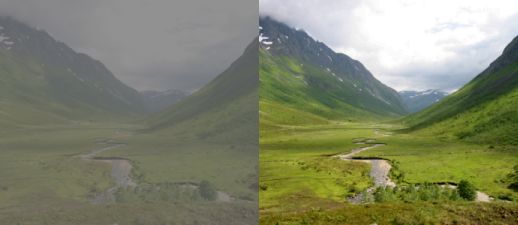```function get_min_max()
min, max = 1000, -1000
for y=0, height-1 do
for x=0, width-1 do
value = get_value(x,y)
if value<min then
min = value
end
if value>max then
max = value
end
end
end
return min,max
end

function remap(v, min, max)
return (v-min) * 1.0/(max-min)
end

function cs_get_rgb(x,y,min,max)
r,g,b = get_rgb(x,y)
r = remap(r, min, max)
g = remap(g, min, max)
b = remap(b, min, max)
return r,g,b
end

function contrast_stretch()
min, max = get_min_max()

for y=0, height do
for x=0, width do
set_rgb(x,y, cs_get_rgb(x,y,min,max))
end
end
flush ()
end

contrast_stretch()
```

## Automatic white balance

In the day of analog point and shoot photography, the white balance of the images were set by the photolab. With digital photography the whitebalance has to either be pre set by the photographer by measurement or guess, or be guessed by algorithms in camera or computer software.

### Grayworld assumption

Assuming that we have a good distribution of colors in our scene, the average reflected color should be the color of the light. If the light source is assumed to be white, we know how much the whitepoint should be moved in the color cube.

The compensation calculated by the gray world assumption is an approximation of the measurement taken by digital still and video cameras by capturing an evenly lit white sheet of paper or similar.

Figure 7.2. grayworld assumption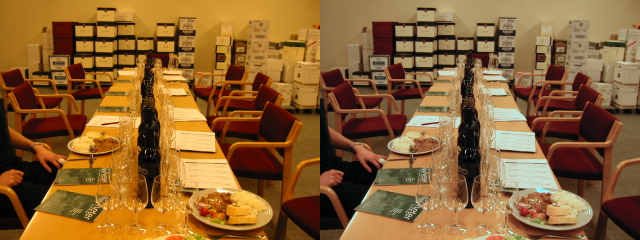```function get_avg_a_b ()
sum_a=0
sum_b=0

-- first find average color in CIE Lab space
for y=0, height-1 do
for x=0, width-1 do
l,a,b = get_lab(x,y)
sum_a, sum_b = sum_a+a, sum_b+b
end
progress(y/height)
end

avg_a=sum_a/(width*height)
avg_b=sum_b/(width*height)

return avg_a,avg_b
end

function shift_a_b(a_shift, b_shift)
for y=0, height do
for x=0, width do
l,a,b = get_lab(x,y)

-- scale the chroma distance shifted according to amount of
-- luminance. The 1.1 overshoot is because we cannot be sure
-- to have gotten the data in the first place.
a_delta = a_shift * (l/100) * 1.1
b_delta = b_shift * (l/100) * 1.1
a,b = a+a_delta, b+b_delta

set_lab(x,y,l,a,b)
end
progress(y/height)
end
flush()
end

function grayworld_assumption()
avg_a, avg_b = get_avg_a_b()
shift_a_b(-avg_a, -avg_b)
end

grayworld_assumption()
```

### Component stretching

Component stretching makes the assumption that either direct reflections, or glossy reflections from surfaces can be found in the image, and that it is amongst the brightest colors in the image. By stretching each R,G and B component to it's full range, as in the section called “Contrast stretching” often leads to a better result than grayworld assumption.

If the image is overexposed, this technique does not work, not having a very reflective object will also bias the results in a non desireable way.

Figure 7.3. component stretching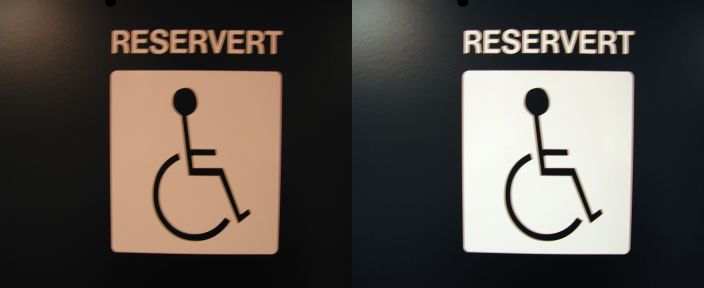```function get_min_max_r ()
min, max = 1000, -1000
for y=0, height-1 do
for x=0, width-1 do
value, temp, temp = get_rgb (x,y)
if value<min then
min = value
end
if value>max then
max = value
end
end
end
return min,max
end

function get_min_max_g ()
min, max = 1000, -1000
for y=0, height-1 do
for x=0, width-1 do
temp, value, temp = get_rgb (x,y)
if value<min then
min = value
end
if value>max then
max = value
end
end
end
return min,max
end

function get_min_max_b ()
min, max = 1000, -1000
for y=0, height-1 do
for x=0, width-1 do
temp, temp, value = get_rgb (x,y)
if value<min then
min = value
end
if value>max then
max = value
end
end
end
return min,max
end

function remap(v, min, max)
return (v-min) * 1.0/(max-min)
end

function cs_get_rgb(x,y,min_r,max_r,min_g,max_g,min_b,max_b)
r,g,b = get_rgb(x,y)
r = remap(r, min_r, max_r)
g = remap(g, min_g, max_g)
b = remap(b, min_b, max_b)
return r,g,b
end

function component_stretch()
min_r, max_r = get_min_max_r ()
min_g, max_g = get_min_max_g ()
min_b, max_b = get_min_max_b ()

for y=0, height do
for x=0, width do
set_rgb(x,y, cs_get_rgb(x,y,min_r,max_r, min_g, max_g, min_b, max_b))
end
end
flush ()
end

component_stretch()
```

## Automatic spatial algorithms

### Local Contrast Enhancement

ACE and Retinex are algorithms that performs color balancing and color enhancements baded on statistical measures of the spatial neighbourhood of each pixel; doing a kind of local contrast stretching. These algorithms aim to model some of the automatic adaptation that happens in the human visual system.

Figure 7.4. lce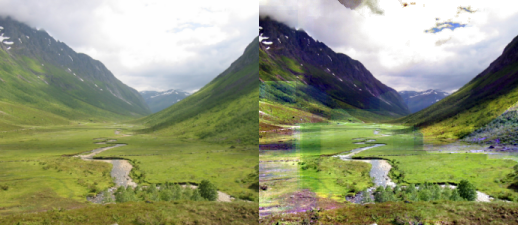```edge_duplicate = 1;

function get_min_max (x0,y0,x1,y1)
min_r, max_r = 1000, -1000
min_g, max_g = 1000, -1000
min_b, max_b = 1000, -1000
for y=y0,y1 do
for x=x0,x1 do
r, g, b= get_rgb (x,y)
if r<min_r then min_r = r end if r>max_r then max_r = r end
if g<min_g then min_g = g end if g>max_g then max_g = g end
if b<min_b then min_b = b end if b>max_b then max_b = b end
end
end
return min_r,max_r,min_g,max_g,min_b,max_b
end

function remap(v, min, max)
return (v-min) * 1.0/(max-min)
end

min_r, max_r, min_g, max_g, min_b, max_b =
r,g,b = get_rgb(x,y)
r = remap(r, min_r, max_r)
g = remap(g, min_g, max_g)
b = remap(b, min_b, max_b)
return r,g,b
end

for y=0, height do
for x=0, width do
end
progress (y/height)
print (y/height)
end
flush ()
end

lce(32)
```

Note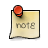This implementation of an local contrast enhancement doesn't really show the potential of this type of algorithms but the implementation is kept simple to be readable and understandable.

## Excercises

### 1. Problems with the Local Contrast Enhancement filter

The artifacts appearing in Figure 7.4, “lce” are due to the sampling of the pixels used to find the maximum and minimum values to do contrast stretching with. How can the filter be improved to give a smoother appearance?